The Fano plane Next: The Cayley-Dickson Construction Up: Constructing the Octonions Previous: Constructing the Octonions

## 2.1 The Fano plane

The quaternions,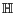, are a 4-dimensional algebra with basis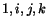. To describe the product we could give a multiplication table, but it is easier to remember that:

•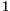is the multiplicative identity,
•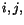andare square roots of -1,
• we have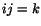,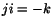, and all identities obtained from these by cyclic permutations of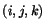.
We can summarize the last rule in a picture: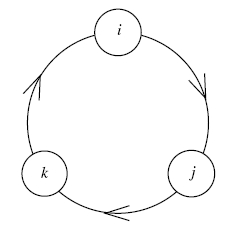When we multiply two elements going clockwise around the circle we get the next one: for example,. But when we multiply two going around counterclockwise, we get minus the next one: for example,.

We can use the same sort of picture to remember how to multiply octonions: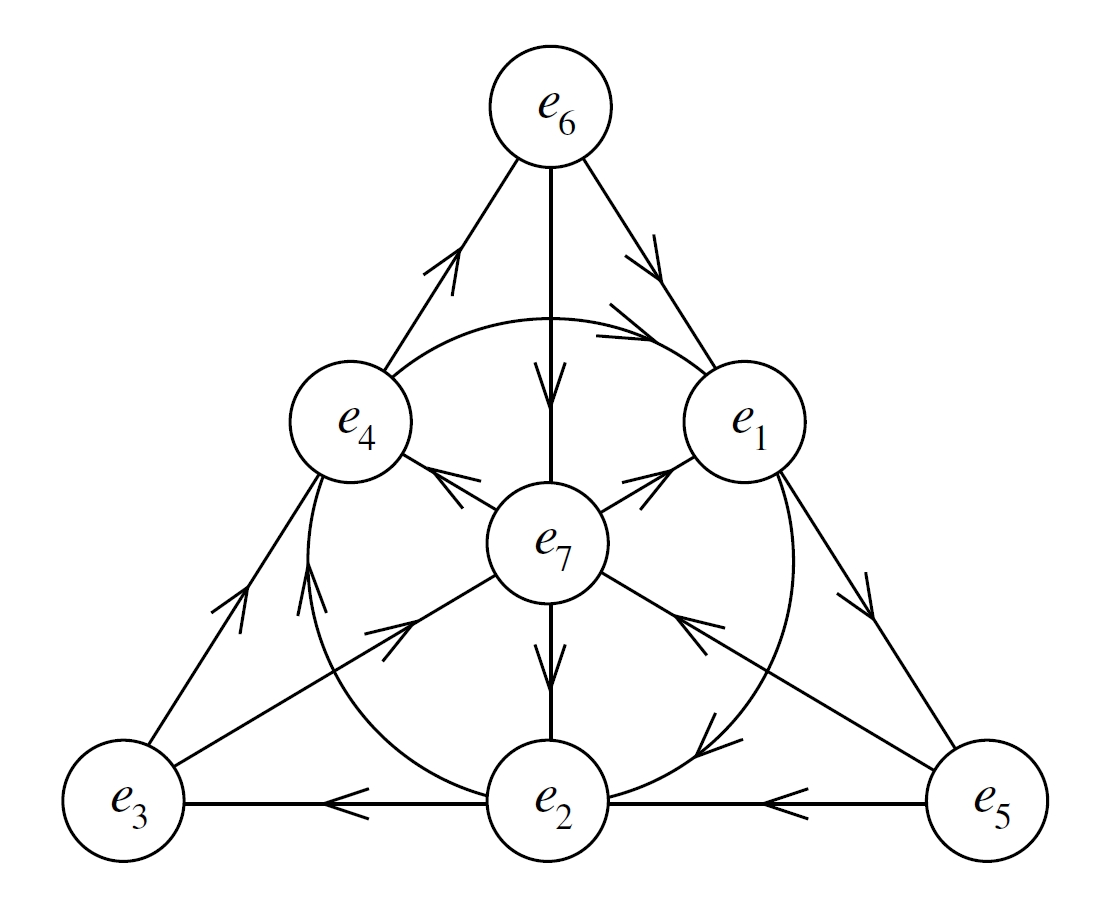This is the Fano plane, a little gadget with 7 points and 7 lines. The 'lines' are the sides of the triangle, its altitudes, and the circle containing all the midpoints of the sides. Each pair of distinct points lies on a unique line. Each line contains three points, and each of these triples has has a cyclic ordering shown by the arrows. If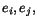and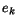are cyclically ordered in this way then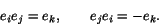Together with these rules:
•is the multiplicative identity,
•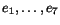are square roots of -1,
the Fano plane completely describes the algebra structure of the octonions. Index-doubling corresponds to rotating the picture a third of a turn.

This is certainly a neat mnemonic, but is there anything deeper lurking behind it? Yes! The Fano plane is the projective plane over the 2-element field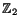. In other words, it consists of lines through the origin in the vector space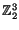. Since every such line contains a single nonzero element, we can also think of the Fano plane as consisting of the seven nonzero elements of. If we think of the origin inas corresponding to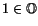, we get the following picture of the octonions: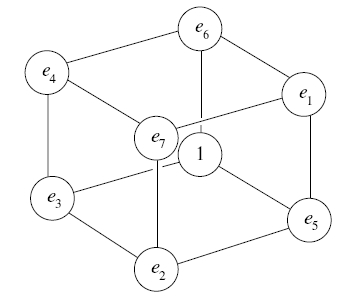Note that planes through the origin of this 3-dimensional vector space give subalgebras of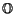isomorphic to the quaternions, lines through the origin give subalgebras isomorphic to the complex numbers, and the origin itself gives a subalgebra isomorphic to the real numbers.

What we really have here is a description of the octonions as a 'twisted group algebra'. Given any group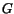, the group algebra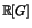consists of all finite formal linear combinations of elements ofwith real coefficients. This is an associative algebra with the product coming from that of. We can use any function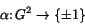to 'twist' this product, defining a new product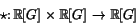by: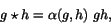where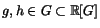. One can figure out an equation involving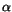that guarantees this new product will be associative. In this case we calla '2-cocycle'. Ifsatisfies a certain extra equation, the product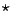will also be commutative, and we calla 'stable 2-cocycle'. For example, the group algebra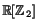is isomorphic to a product of 2 copies of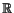, but we can twist it by a stable 2-cocycle to obtain the complex numbers. The group algebra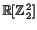is isomorphic to a product of 4 copies of, but we can twist it by a 2-cocycle to obtain the quaternions. Similarly, the group algebra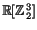is a product of 8 copies of, and what we have really done in this section is describe a functionthat allows us to twist this group algebra to obtain the octonions. Since the octonions are nonassociative, this function is not a 2-cocycle. However, its coboundary is a 'stable 3-cocycle', which allows one to define a new associator and braiding for the category of-graded vector spaces, making it into a symmetric monoidal category . In this symmetric monoidal category, the octonions are a commutative monoid object. In less technical terms: this category provides a context in which the octonions are commutative and associative! So far this idea has just begun to be exploited.

Next: The Cayley-Dickson Construction Up: Constructing the Octonions Previous: Constructing the Octonions